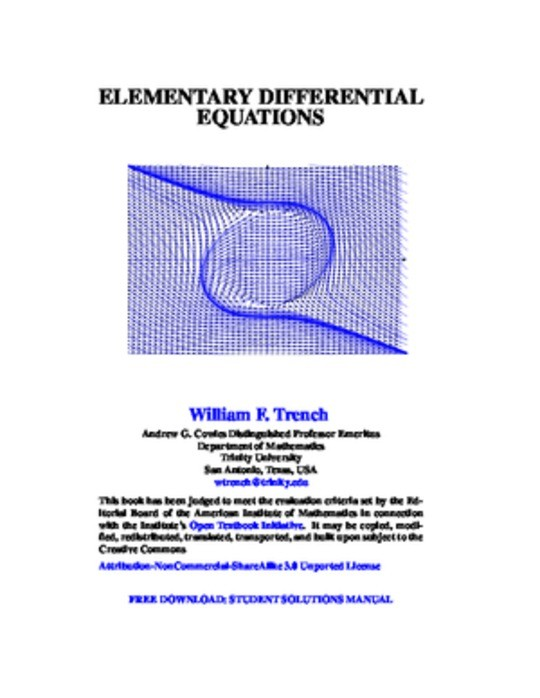Unknown
Science & Math
Elementary Differential Equations
Free
Description
Contents
Reviews
Language
English
ISBN
Unknown
Preface
Chapter 1 Introduction
Section 1.1 Some Applications Leading to Differential Equations
Section 1.2 Basic Concepts
Section 1.3 Direction Fields for First Order Equations
Chapter 2 First Order Equations
Section 2.1 Linear First Order Equations
Section 2.2 Separable Equations
Section 2.3 Existence and Uniqueness of Solutions of Nonlinear Equations
Section 2.4 Transformation of Nonlinear Equations into Separable Equations
Section 2.5 Exact Equations
Section 2.6 Exact Equations
Chapter 3 Numerical Methods
Section 3.1 Euler's Method
Section 3.2 The Improved Euler Method and Related Methods
Section 3.3 The Runge-Kutta Method
Chapter 4 Applications of First Order Equations
Section 4.1 Growth and Decay
Section 4.2 Cooling and Mixing
Section 4.3 Elementary Mechanics
Section 4.4 Autonomous Second Order Equations
Section 4.5 Applications to Curves
Chapter 5 Linear Second Order Equations
Section 5.1 Homogeneous Linear Equations
Section 5.2 Constant Coefficient Homogeneous Equations
Section 5.3 Nonhomogeneous Linear Equations
Section 5.4 The Method of Undetermined Coefficients I
Section 5.5 The Method of Undetermined Coefficients II
Section 5.6 Reduction of Order
Section 5.7 Variation of Parameters
Chapter 6 Applications of Linear Second Order Equations
Section 6.1 Spring Problems I
Section 6.2 Spring Problems II
Section 6.3 The RLC Circuit
Section 6.4 Motion Under a Central Force
Chapter 7 Series Solutions of Linear Second Equations
Section 7.1 Review of Power Series
Section 7.2 Series Solutions Near an Ordinary Point I
Section 7.3 Series Solutions Near an Ordinary Point II
Section 7.4 Regular Singular Points Euler Equations
Section 7.5 The Method of Frobenius I
Section 7.6 The Method of Frobenius II
Section 7.7 The Method of Frobenius III
Chapter 8 Laplace Transforms
Section 8.1 Introduction to the Laplace Transform
Section 8.2 The Inverse Laplace Transform
Section 8.3 Solution of Initial Value Problems
Section 8.4 The Unit Step Function
Section 8.5 Constant Coeefficient Equations with Piecewise Continuous Forcing Functions
Section 8.6 Convolution
Section 8.7 Constant Coefficient Equations with Impulses
8.8 A Brief Table of Laplace Transforms
Chapter 9 Linear Higher Order Equations
Section 9.1 Introduction to Linear Higher Order Equations
Section 9.2 Higher Order Constant Coefficient Homogeneous Equations
Section 9.3 Undetermined Coefficients for Higher Order Equations
Section 9.4 Variation of Parameters for Higher Order Equations
Chapter 10 Linear Systems of Differential Equations
Section 10.1 Introduction to Systems of Differential Equations
Section 10.2 Linear Systems of Differential Equations
Section 10.3 Basic Theory of Homogeneous Linear System
Section 10.4 Constant Coefficient Homogeneous Systems I
Section 10.5 Constant Coefficient Homogeneous Systems II
Section 10.6 Constant Coefficient Homogeneous Systems III
Section 10.7 Variation of Parameters for Nonhomogeneous Linear Systems
A Brief Table of Integrals